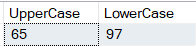# Built-in String Functions in SQL Server

## Built-in String Functions in SQL Server

In this article, I am going to discuss the Built-in string functions in SQL Server with examples. The Functions in SQL server broadly divided into 2 types

1. Built-in function
2. User-Defined Functions

Built-In string functions in SQL Server are used with SQL SELECT expressions to calculate values and manipulate the data. These built-in functions can be used anywhere, where expressions are allowed.

##### What are Built-In Functions?

In SQL Server a built-in function is a piece of code that takes one or more inputs and returns a value.  An example of a built-in function is ABS(), which when given a value calculates the absolute (non-negative) value of the number.

Note: SQL built-in functions may or may not take parameters but always returns a value.

There are several built-in functions available in SQL Server. In this article, we will discuss the most common use of built-in string functions in SQL Server.

###### ASCII(Character_Expression)

The ASCII function returns the ASCII code that represents a specific character.
To find the ASCII Code of the capital letter ‘A’

Example: Select ASCII(‘A’)

Output: 65

This function is commonly used for comparing characters without knowing whether they are in the upper case or lower case. Uppercase and lower case letters translate into different ASCII values

Let’s see with an example.

Select ASCII(‘A’) as UpperCase, ASCII(‘a’) as LowerCase

OUTPUT:###### CHAR(Integer_Expression)

This function converts an integer ASCII code to a character. That means the CHAR function does the opposite of the ASCII function. The Integer_Expression should be between 0 and 255.

##### Printing uppercase alphabets using CHAR() function:
```DECLARE @Number int
SET @Number = 65
WHILE(@Number <= 90)
BEGIN
PRINT CHAR(@Number)
SET @Number = @Number + 1
END
```

Note: The while loop will become an infinite loop if you forget to include the following line.

Set @Number = @Number + 1

##### Printing lowercase alphabets using CHAR() function:
```DECLARE @Number int
SET @Number = 97
WHILE(@Number <= 122)
BEGIN
PRINT CHAR(@Number)
SET @Number = @Number + 1
END
```
##### Another way of printing lower case alphabets using CHAR() and LOWER() functions.
```DECLARE @Number int
SET @Number = 65
WHILE(@Number <= 90)
BEGIN
PRINT LOWER(CHAR(@Number))
SET @Number = @Number + 1
END
```
###### LTRIM(Character_Expression)

This function returns a character expression after it removes the leading blanks. That means it removes blanks on the left-hand side of the given character expression.

Example: Removing the 3 white spaces on the left-hand side of the ‘   Hello’ string using LTRIM() function.

Select LTRIM(‘   Hello’)

Output: Hello

###### RTRIM(Character_Expression)

This function returns a character string after truncating all trailing blanks. That means it removes blanks on the right-hand side of the given character expression.

Example: Removing the 3 white spaces on the left-hand side of the ‘Hello   ‘ string using RTRIM() function.

Select RTRIM(‘Hello   ‘)

Output: Hello

If you want to remove white spaces on either side of the given character expression, then you need to use LTRIM() and RTRIM() as shown below.

Select LTRIM(RTRIM(‘   Hello   ‘))

Output: Hello

###### LOWER(Character_Expression)

This function returns a character expression after converting all the uppercase character data to lowercase. That means it converts all the characters in the given Character_Expression to lowercase letters.

ExampleSelect LOWER(‘CONVERT This String Into Lower Case’)

Output: convert this string into lower case

###### UPPER(Character_Expression)

This function returns a character expression with lowercase character data converted to uppercase. That means it converts all the characters in the given Character_Expression to uppercase letters.

ExampleSelect UPPER(‘CONVERT This String Into upperCase’)

Output: CONVERT THIS STRING INTO UPPERCASE

###### REVERSE(‘Any_String_Expression’)

This function returns the reverse of a character expression. That means it reverses all the characters in the given string expression.

ExampleSelect REVERSE(‘ABCDEFGHIJKLMNOPQRSTUVWXYZ’)
Output: ZYXWVUTSRQPONMLKJIHGFEDCBA

###### LEN(String_Expression)

This function returns the number of characters, instead of the number of bytes, of the specified string expression, excluding trailing blanks. That means it returns the count of total characters in the given string expression, excluding the blanks at the end of the expression.

ExampleSelect LEN(‘ Functions   ‘)

Output: 10

###### LEFT(Character_Expression, Integer_Expression)

This function returns the left part of a character string with the specified number of characters. That means it returns the specified number of characters from the left-hand side of the given character expression.
Example
Select LEFT(‘ABCDE’, 3)

Output: ABC

###### RIGHT(Character_Expression, Integer_Expression)

This function returns the right part of a character string with the specified number of characters. That means it returns the specified number of characters from the right-hand side of the given character expression.
Example: Select RIGHT(‘ABCDE’, 3)

Output: CDE

###### CHARINDEX(‘Expression_To_Find’, ‘Expression_To_Search’, ‘Start_Location’)

This function returns the starting position of the specified expression in a character string. The Start_Location parameter is optional.

Example: In this example, we get the starting position of ‘@’ character in the email string ‘sara@aaa.com’.

Select CHARINDEX(‘@’,’hina@aaa.com’,1)
Output: 5

###### SUBSTRING(‘expression’, ‘Start’, ‘Length’)

As the name, suggests, this function returns substring (part of the string), from the given expression. You specify the starting location using the ‘start’ parameter and the number of characters in the substring using the ‘Length’ parameter. All 3 parameters are mandatory.

Example: Display just the domain part of the given email ‘info@dotnettutorials.net’.

Select SUBSTRING(‘info@dotnettutorials.net’,6, 19)

Output: dotnettutorials.net

In the above example, we have hardcoded the starting position and the length parameters. Instead of hard-coding, we can dynamically retrieve them using CHARINDEX() and LEN() string functions as shown below.

Example:
Select SUBSTRING(‘info@dotnettutorials.net’,(CHARINDEX(‘@’, ‘info@dotnettutorials.net’) + 1),

(LEN(‘info@dotnettutorials.net’) – CHARINDEX(‘@’,’info@dotnettutorials.net’)))

Output: dotnettutorials.net

1.